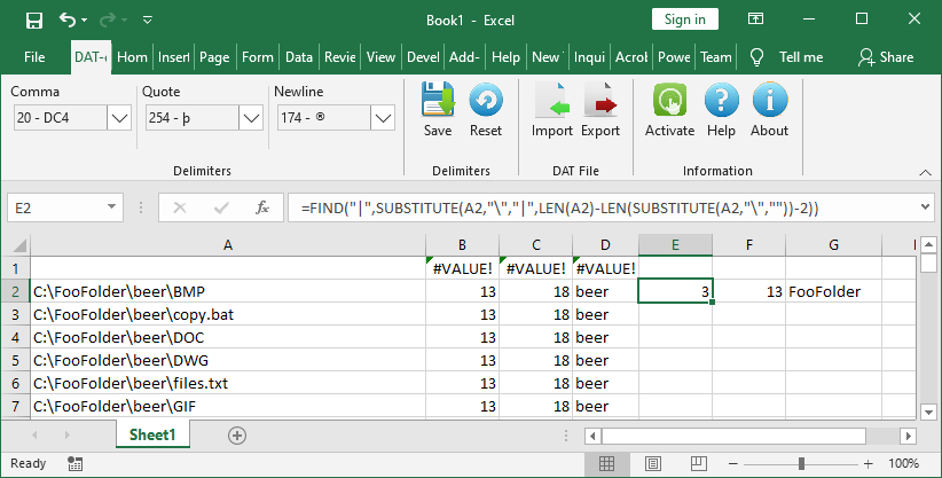Search

# Excel formula to extract folder from file path

You can use three Excel formulas to extract a folder from a file path.

In this example we have file paths listed in column A.

1. In column B enter this formula:

=FIND("|",SUBSTITUTE(A1,"\","|",LEN(A1)-LEN(SUBSTITUTE(A1,"\",""))-1))

. . . this finds the position of the next to last slash.

2. In column C enter this formula:

=FIND("\",A1,B1+1)

. . . this finds the position of the last slash

3. In column D, the MID - middle - formula pulls the data between the positions identified in the two columns to the left:

=MID(A2,B2+1,(C2-B2)-1)You can go one folder back further in the file path by changing the formula to get the position of the next slash:

=FIND("|",SUBSTITUTE(A2,"\","|",LEN(A2)-LEN(SUBSTITUTE(A2,"\",""))-2))

. . . at the end the 1 is changed to a 2. You can re-use the formula from step 1, and then simply repoint the MID formula to the new range results.